Home | | Maths 2nd Std | Addition

## Chapter: 2nd Maths : Term 1 Unit 2 : Numbers

Teacher’s Note: Teacher can help the students to try adding of numbers using variety of materials.

Recall

Kanmani, Asha and Prashanth used ice sticks for their craft work. Find the total number of sticks used by each of them from the picture given below and write them in the blue box.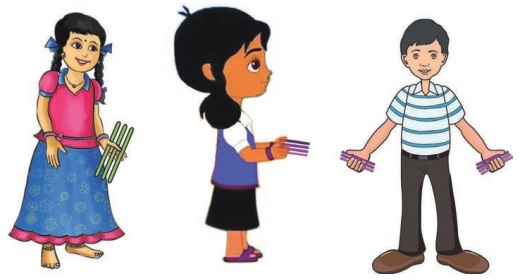Teacher’s Note: Teacher can help the students to try adding of numbers using variety of materials.

Travel Through

A journey to the zoo

There are 20 members in the zoo train. Raj counts the members entering into the zoo train at each station in the zoo.Keywords: Count, Add, Total, Combine, Altogether

2 children boarded the train after visiting the white tigers.

20 + 2 = 22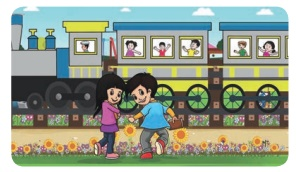From the aquarium, 5 children boarded the train.

22 +  5 = 27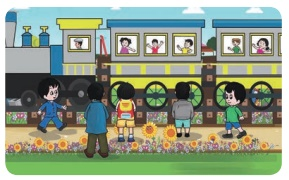2 children boarded the train at the butterfly park.

27 + 2 = 29Teacher’s Note: Teacher may extend the situation by varying the number of children who boarded into the train and encourage the students to find the total.

Learn

1. Muthu bought 32 lemons and Kannan bought 5 lemons. Find the total number of lemons bought by them.2. Abi plucked 24 guavas, Jesi plucked 33 guavas. How many guavas did Abi and Jesi pluck in all?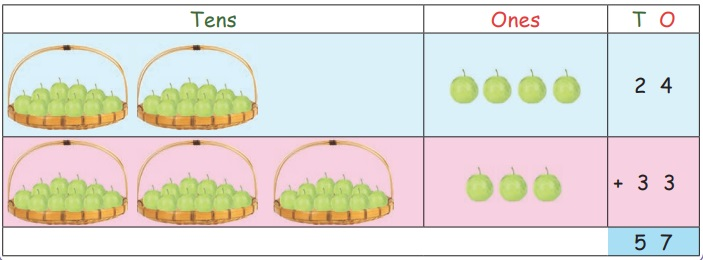Practice

1. A balloon seller has 23 pink balloons and 4 yellow balloons. How many balloons did he has totally?2. In an inter school competition, a school had won 30 gold medals and 21 silver medals. Find the total number of medals won by the school.3. Reena bought 30 pens from the shop. Next day, she purchased 50 more pens. Count and write the total number of pens she had.4. Mani has 54 pencils, Benny has 21 pencils and Malini has 23 pencils. How many pencils do they have in total?Learn

1) Add using fingers : 24 + 324 + 3 = 27

2) Add using number line : 14 + 5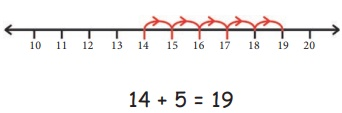14 + 5 = 1932 + 24 + 13 = 69

4) Add using abacus : 26 + 6126 + 61 = 87

Practicei) 34 + 35 =  69

ii) 13 + 2 + 1 =  16

iii) 14 + 10 + 2 =  26

iv) 34 + 30 + 3 =  67

v) 30 + 26 + 40 =  96

vi) 45 + 23 + 21 =  89

Try This

Colour the tens and ones for given numbers 43 and 25. Find the sum: 43 + 25

Add the ones of the given number by colouring single beads. Add the tens of the given number by colouring strips of ten beads.Pleasure Time

Complete the circles on the right by adding each of the numbers on the left with 10 and with 14. One is done for you.a)

20 + 10 = 30

25 + 10 = 35

36 + 10 = 46

48 + 10 = 58

b)

32 + 14 = 46

40 + 14 = 54

53 + 14 = 67

65 + 14 = 79

Know more

Both are same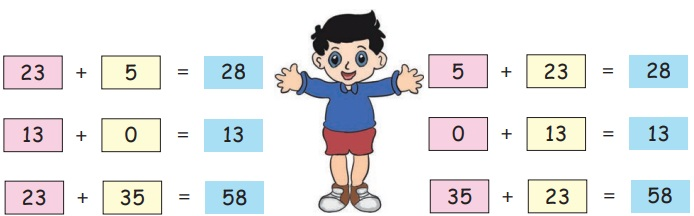23 + 5 = 28                5 + 23 = 28

13 + 0 = 13                0 + 13 = 13

23 + 35 = 58             35 + 23 = 58

Activity

Divide the class into 2 groups and give 100 sticks (bundles of 10 sticks and few single sticks) to each group.

* Teacher shall give two numbers to add. For example, 42 and 56

* The first group shall take 40 + 2 sticks and the second group shall take 50 + 6 sticks.

* Both the groups shall combine the sticks. Count the bundles of sticks and single sticks separately and fi nd the total.

* Teacher can instruct the groups to add the single sticks first, write the number below the ones place and then add the bundle of sticks and write the number below the tens place.

Mental Maths

1. 30 children are lined up to jump using a skipping rope. If 20 more children join them, how many children are there in total?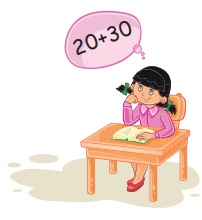30 + 20 = 50Ans : Total  Children = 50

2. In a park, Suji counted 12 children and 15 adults. How many people did she count in total?12 + 15 = 27

3. A fisherman caught 22 fishes in the morning and 12 fishes in the afternoon. How many fishes did he catch totally?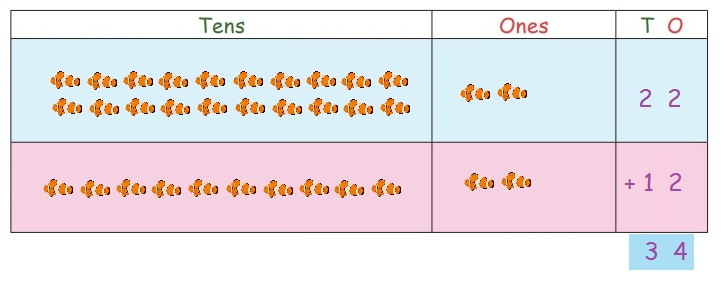22 + 12 = 34

Ans : Total  fishes = 34

4. There are 15 boys and 24 girls in a class room. How many students are there altogether?15 + 24 = 39

Ans : Total  students = 39

5. In a bird sanctuary, there are 33 parrots and 15 peacocks. How many birds are there in total?33 + 15 = 48

Ans : Total birds = 48

Tags : Numbers | Term 1 Chapter 2 | 2nd Maths , 2nd Maths : Term 1 Unit 2 : Numbers
Study Material, Lecturing Notes, Assignment, Reference, Wiki description explanation, brief detail
2nd Maths : Term 1 Unit 2 : Numbers : Addition | Numbers | Term 1 Chapter 2 | 2nd Maths